# Javascript Data Types

Javascript Data Types

If you are already familiar with data, the data is some kind of information. It can be anything, like a number, or someone’s name, email address, home address, company name, salary, etc.

Now you have already understood that we use variables to store the data. Now it’s time to learn what are the types of data that we are going to deal with.

So, as mentioned above, data is some kind of information. It can be a string(set of characters), a number(decimal or integer), or boolean(true/false), null(nothing), or undefined(for a variable that has not been assigned any value).

## Javascript Data Types

These are all kinds of Primitive data types(basic data types).

There are some other things like objects, which we are going to discuss as we move forward.

Also, we are going to see an operator, which is a type of, use in which, we are going to get the type of data that we are using.

First of all, understand that there are different types of data that we are dealing with.

Let’s first have a look at storing number data to the variable. Have a look at the below program, and after that, we will have a brief explanation about it as well.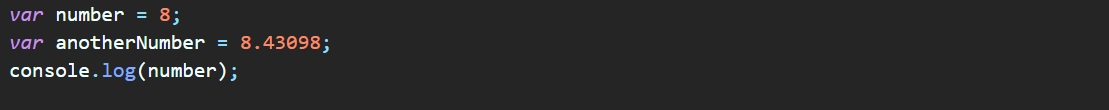Well, the above code is just some JavaScript. Other than that, the HTML file is already created and we have given this JavaScript file as the source for that HTML file. Since majorly, we are going to deal with JavaScript here, we would be writing our JavaScript into a JavaScript file.

Now, let’s have an explanation for the above-written lines. We are already familiar with how can we create variables. We are just creating some variables, and assigning some value to them. That value has some type. So, depending upon what value are we giving, the type will be decided. So, for the above 2 variables that we have created, the values are numbers, so a type is also a number. You can verify it using the type of operator. Here is how you can use that –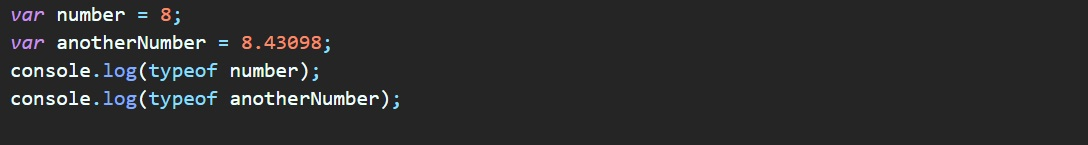If you move to the console with this file, the output as you can find would be a number, for both the above-created variables. You can have some bigger numbers too. For a number, the max value that we can have comes out to bee 1.7976931348623157e+308. This is a huge number actually. While we have the max value as the above-specified number, the safe value that we have is 9007199254740991 (MAX_SAFE_INTEGER), or we can say approximately 9 quadrillions.

Well, while we are within the max safe integer, the operations that we are going to perform on numbers, would be accurate and correct. But beyond that, we need to use something called as BigInt, through which, we can operate on numbers beyond the max safe integer. You can also try storing some other data as well, into the program. It is pretty easy, and often while doing some programs, we would also be using different types of data.

You can refer to the below code to understand the data types in a better way –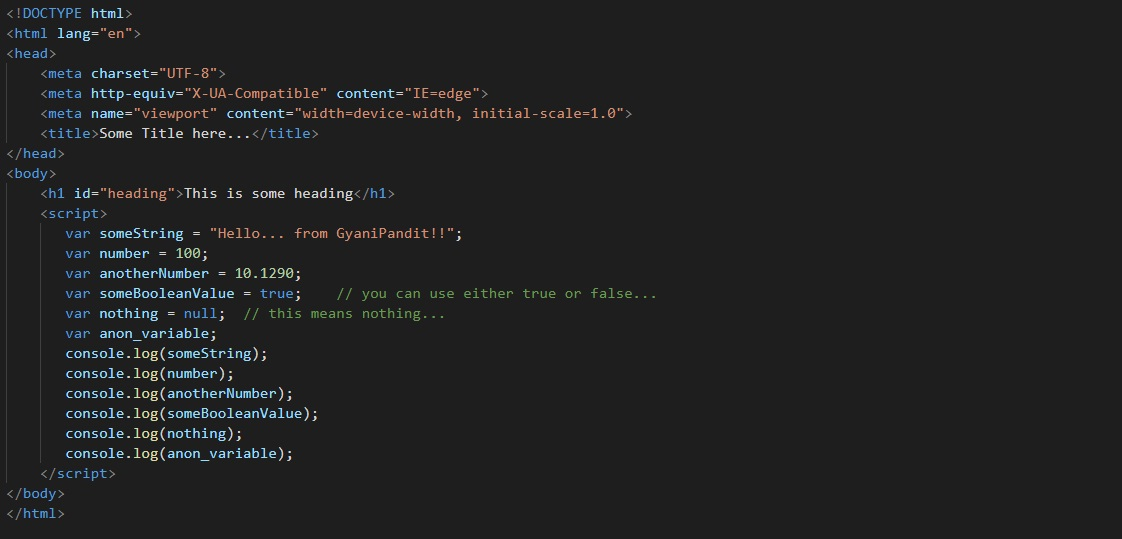Have a look at the output now →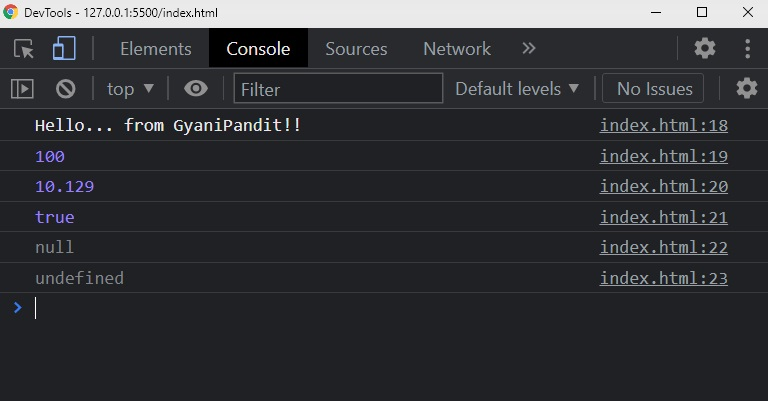As you can see, the first data is a string. The next two pieces of data are the numbers(one is an integer, and another is a floating-point, but both are numbers!). Next, we have a boolean type data(which means, only true or false), the next is null(this means the intentional absence of some object value), and the last is undefined(when we have declared the variable, but no value).

### Using the type of operator to determine the type of the variable you created →

Well, you know that we can create a variable for different data types. Now, using the type of operator, we can determine the data type of some variables. Let’s have a look at how can we do this. Well, we are going to be modifying the above-discussed program, to understand the type of operator –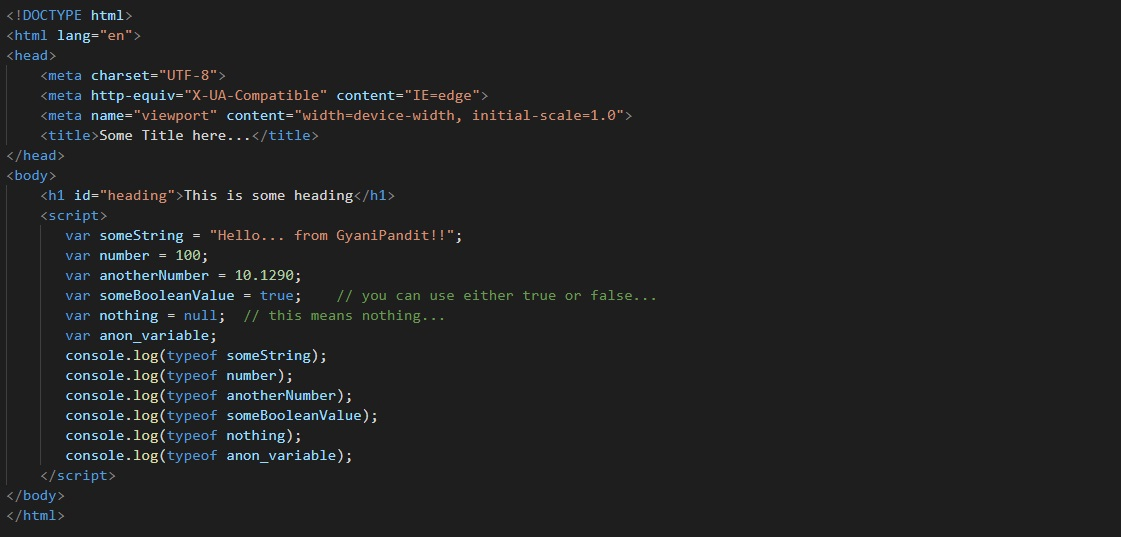Have a look at the output now. We have got the type of variables that we have made above –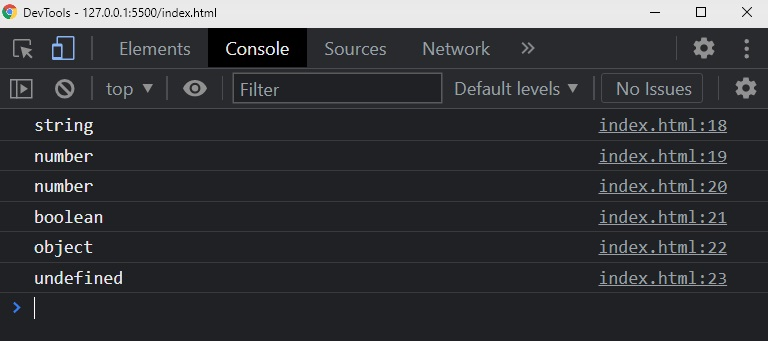As you can see, we got some different outputs here. With this, we can understand some different data types in JavaScript. We will become used to these, once we keep on using them. We can use the type of operator often, to check the types of different data that we have.